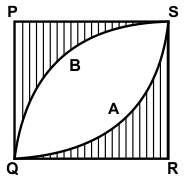# CREST Mathematics Olympiad Class 10 Sample Papers

REGISTER NOW

## Syllabus:

Section 1: Real Numbers, Polynomials, Pair of Linear Equations in Two Variables, Quadratic Equations, Arithmetic Progressions, Triangles, Coordinate Geometry, Introduction to Trigonometry, Some Applications of Trigonometry, Circles, Constructions, Areas Related to Circles, Surface Areas and Volumes, Statistics, Probability.

Achievers Section: Higher Order Thinking Questions - Syllabus as per Section 1

 Q.1 The average weight of A, B and C is 84 kg. If D joins the group, the average weight of the group becomes 80 kg. If another man E who weighs 3 kg more than D replaces A, then the average of B, C, D and E becomes 79 kg. What is the weight of A ?
 Q.2 30% of the items were sold at a profit of 40% while the remaining were sold at x% loss. If the overall loss is 10%, find the value of x:
 Q.3 If [(x - a)/(b + c)] + [(x - b)/(c + a)] + [(x - c)/(a + b)] = 3, then find the value of x:
 Q.4 The volume of a pyramid whose base is an equilateral triangle is 12 cm3. If the height of the pyramid is 3√3 cm, then find the length of each side of the base:
 Q.5 Which term is numerically greatest term in the expansion of (3 + 2x)49, when x = 1/5?
 Q.6 W borrowed a certain sum of money from X at the rate of 10% per annum under simple interest and lent one-fourth of the amount to Y at 8% per annum under simple interest and the remaining amount to Z at 15% per annum under simple interest. If at the end of 15 years, W made profit of ₹5850 in the deal, then find the sum that W had lent to Z:
 Q.7 How many 5-digit odd numbers can be formed using the digits 2, 3, 5, 7, 8, and 9 if every digit can occur at most once in any number?
 Q.8 If the coefficients of rth term and (r + 1)th term in the expansion of (1 + x)20 are in the ratio 1:2, what is the value of r?
 Q.9 In the given figure, PQRS is a square of side 7√2 cm. With P, R as centres and PQ as radius, the arcs QAS and QBS are drawn, respectively. Find the area of the shaded region (in cm2):Q.10 If cos x/(1 + cosec x) + cos x/(cosec x - 1) = 2, then which one of the following is one of the values of x?

Sample PDF of CREST Mathematics Olympiad for Class 10: